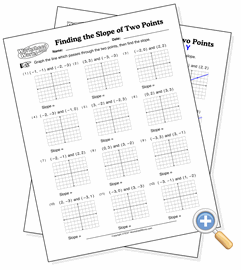# Finding the Slope of Two PointsFind the slope of lines drawn graphed from two points

In this worksheet, students graph lines from two points, then find the slope. After drawing the line, they can count lines on the grid to find the slopes.